Let’s face it, interconnects are the major bottleneck in communication between electronic components. 25-30 Gbps per one serial channel is becoming the mainstream, but what about 100 Gbps? Such data rates and even much higher can be generated and transmitted on a small scale on chip, but what about getting it through packaging and PCB interconnects to the other components?  Is it even possible to design interconnects transparent to the signal with predictable behavior for those data rates? With existing mass manufacturing technology and the current state of the mainstream EDA modeling software, it is difficult and in many cases not possible to achieve the analysis to measurement correlation even for 28 Gbps  and is exponentially challenging at the higher data rates.

What are the obstacles? Lossy and dispersive materials and violation of interconnect localization in the existing PCB and packaging manufacturing process are probably the top two obstacles. To build predictable models, first of all, we need to understand the physical properties of the materials and to use extremely broadband models for dielectrics and conductors. Without them, even 3D electromagnetic analysis of interconnects will not correlate with the measurements that introduces high risk into the interconnect design cycle. This paper explains physics of the conductor-related loss and dispersion effects that lead to signal degradation. In particular, physics of the skin-effect on flat and rough conductor surfaces are explained in details and with examples. We will discuss the conductor effects mostly in context of signal transmission with the quasi-TEM waves in transmission lines with two or more conductors.

Analysis of such interconnects with 3D and full-wave tools does not automatically guarantees that all conductor-related effects are properly accounted for. For instance, solvers originally developed for microwave applications are optimized for analysis of relatively narrow-band systems and for frequencies where skin-effect is well developed. Such models are not valid at low and intermediate frequencies where there is no skin-effect or skin-effect is not developed yet and the models have to include those frequencies for digital applications. As the result, a broadband models of interconnects may show non-physical behavior of extracted inductance and resistance at low and intermediate frequencies and violate the causality requirements. In other words, the transition to skin effect in traces and metal planes are usually neglected in 3D EM tools in general. The frequency-dependent skin-effect is especially difficult to approximate with high accuracy in 3D full-wave time-domain tools – such approximation is usually bandwidth-limited. Skin-effect on rough conductor surface may have dramatic consequences on the signal degradation, increasing the conductor-related losses substantially. Though, just a few electromagnetic tools have advanced conductor roughness models that correlate with the measurements for PCBs.

PCB and packaging interconnects usually use conductive traces, wires and planes to connect electronic components. We will not discuss conductor-less dielectric waveguides or optical interconnects here. The conductor-based interconnects or waveguide structures in general have multiple advantages and can potentially support transmission with data rates up to Terabit per second and much higher. Though, to achieve that, we have to understand at least macroscopic electromagnetics of the conductors.

Word conductor here is used for two things: conductor as the material (copper for instance or other metal) and conductor as the conductive element of interconnects or corresponding transmission line model (signal or reference conductor such as trace or plane for instance). It is usually obvious which meaning of the conductor word is used from the context.

### Conductors as materials

Let’s start with the formal definition of conductor. Conductors in general are materials that allow flow of free charges. Macroscopic movement of free charges is described by current density term Jfree in Ampere’s law of the Maxwell’s equations shown in Fig. 1 (see Ampere’s law in the context of complete set of equations in Appendix 1). Let’s restrict our attention here to just Linear Time Invariant (LTI) and isotropic materials (see definitions in Appendix 2). This assumption is suitable for practically all conductors used to manufacture PCB and packaging interconnects. When the electric field, that is basically a force on a unit charge, is applied to a material with free charges inside, charges start moving in the direction of the electric field as is illustrated in Fig. 1.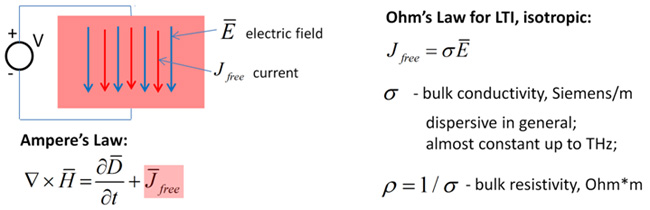Fig. 1. Translational motion of charges in the electric field described by Ampere’s and Ohm’s laws.

Note, that the charges move very slowly comparing to the signal propagation speed – about 5 mm/s in copper at room temperature in electric field 1 V/m . The translational movement of the charges is described by the macroscopic current density parameter measured in A/m^2 (SI units are used here). The total current through the conductor is equal to the current density integrated over the cross-section of the conductor (it is just the product of current density and the conductor cross section area at DC). In general, the current density depends on the electric field, temperature and may be some other parameters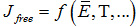. However, for LTI isotropic conductors, the relationship between the electric field strength and the current density is particularly simple and is expressed by the first constitutive or material equation called Ohm’s law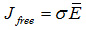It is simply linear dependency for practically all cases relevant to interconnects. Here, σ is bulk  conductivity measured in Siemens/m or 1/Ohm*m. The conductivity is dispersive in general (it is frequency-dependent), but almost constant for good conductors up to THz frequency range. The inverse of the bulk conductivity is the bulk resistivity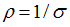, measured in Ohm*m.

Practically all existing materials have free charges and related to that non-zero value of bulk conductivity or resistivity. The number of free charges or values of the conductivity or resistivity can be used to separate all materials into good dielectrics or insulators and good conductors as illustrated in Fig. 2 [3,4].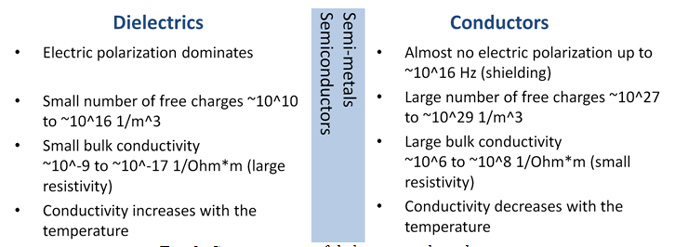Fig. 2. Common traits of dielectrics and conductors.

There are materials on the border between good dielectrics and good conductors, but they are outside of the scope of this paper. Impact of dielectric polarization behavior on the signal degradation will be discussed in another paper of “How Interconnects Work” series. The ideal insulator or dielectric has zero bulk conductivity or infinite bulk resistivity. It is property of only vacuum and there are no such materials. Bulk conductivity of one of the best solid dielectric quartz is around 10^-17 S/m. Conductivity of the isolative materials used to construct PCBs and packaging interconnects may range from 10^-10 to 10^-12 (glass) S/m.

The ideal conductor has infinite bulk conductivity or zero resistivity. It is an abstract or non-existent material and should not be confused with the super-conductors that have extremely small resistivity, but also have specific temporal dispersion related to the kinetic inductance of the charge pairs. Conductivity of conductors used in PCB and packaging interconnects may range between 5*10^6 (lead) to 6.1*10^7 S/m (silver, the best conductor). Bulk conductivity of annealed copper is about 5.8*10^7 S/m. That is reciprocal of resistivity 1.724*10^-8 Ohm*m. It is often observed that the conductivity of copper used to manufacture interconnects may be slightly smaller (larger resistivity) due to peculiarities of the manufacturing process and inhomogeneity. However, it may be also related to uncertainty in measurements of conductor cross-section that comes from uncertainty of the conductor boundaries due to large roughness (discussed later).

### Electromagnetic fields and currents in conductors

Conductive traces and planes on PCBs can be formally modeled as a composition of segments of transmission lines with two or more conductors and discontinuity models [5,6]. The multiconductor transmission line models are usually constructed with static, quasi-static or electromagnetic field solvers. The multiconductor transmission lines with mostly Transverse Electric and Magnetic (quasi-TEM) waves are formally described with Telegrapher’s equations with high accuracy [7,8]. A field solver extracts admittance and impedance per unit length (p.u.l.) and modal parameters such as modal complex propagation constant and characteristic impedance for further analysis of line segments and complete channel. We need to understand the effects of the conductors on the complex propagation constant and characteristic impedance of the waves used to transmit the signal. The real part of the complex propagation constant is the attenuation. The signal losses are higher at higher frequencies and in t-lines with higher attenuation. The imaginary part of the complex propagation constant defines the phase for the transmitted signal. There is no dispersion if it is linear function of frequency – such ideal connection has flat phase or group delay. Any deviation of phase from linearity distorts the signal (different signal harmonics have different delay). The conductor-related effects are mostly included into the p.u.l. impedance part of the Telegrapher’s equations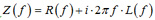. The real part of the impedance R(f) is frequency-dependent p.u.l. resistance of the conductors. The imaginary part is inductive with the inductance that can be further separated into two parts with external and conductor internal frequency-dependent p.u.l. inductance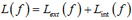. It is convenient to use the resistance and internal conductor inductance for qualitative analysis of the conductor-related effects.

Quasi-TEM waves propagating in multiconductor lines have electric and magnetic field components predominantly tangential to the line cross-section and the signal is transmitted along the line by these fields outside of the conductors. The fields may have components along the line if there is inhomogeneous or multilayered dielectrics and also in the case of lossy or non-ideal conductors discussed here. It is very important to understand that the signal energy is transmitted by the electromagnetic fields in dielectrics (or vacuum) and not by the currents in the conductors. Mathematically, fields inside the conductors can be described with very high accuracy with the Maxwell’s equations without the displacement term (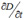in the Ampere’s law) as a magneto-quasi-static or diffusion problem. It is mostly the electric field directed along the conductor causes the current flow in the conductor according to the Ohm’s law. Remember that the electric field is the force on the unit charge. The current flow generates the magnetic field circulating around the conductor and inside the conductor as described by the Ampere’s law and illustrated in Fig. 3. On the other hand, according to the Faraday’s law, changes in of the magnetic field cause electric field directed opposite to the current flow and opposite to the electric field that caused the current. The opposite electric field is very small at low frequencies, but grows with the frequency. As the result, starting from some frequencies we can observe the electric field and the related by Ohm’s law current density cancellation effect at the conductor interior as illustrated in Fig. 3. At sufficiently high frequencies electromagnetic field inside the conductor close to the surface can be approximated as the plane wave transmitting the energy inside the conductor as also illustrated in Fig. 3. This is the base for surface impedance boundary conditions or SIBC used in most of the electromagnetic field solver. It produces non-uniform conductor current distribution as qualitatively illustrated in Fig. 3 for a simple case of rectangular conductor. Parameter δs in the complex exponential function for the electric field or current density in the conductor in Fig. 3 is called skin depth and can be calculated as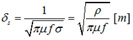. Here is bulk conductivity σ in S/m, ρ is bulk resistivity in Ohm*m, μ is magnetic permeability in H/m and f is frequency in Hz.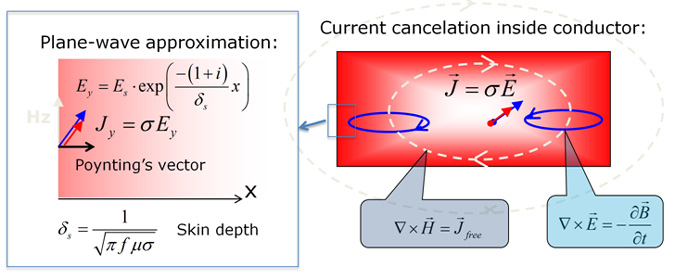Fig. 3. Skin effect. Electric field and current cancelation inside the conductor at high frequency (right) and exponential plane wave approximation of fields and current density inside conductor (left). The rectangular conductor in a t-line with signal propagating along the Y-axis with cross section in the XZ-plane.

The skin depth δs becomes smaller at higher frequencies and eventually becomes much smaller than the conductor cross-section and we can call this state as well-developed skin-effect. As the formula predicts, the energy inside the conductor is transmitted into the direction normal to the conductor surface and not in the direction of signal propagation! It may be difficult to comprehend, but the conductor interior with all those moving charges has nothing to do with the transmission of the signal energy along the line – the conductors just suck out the energy. The conductors form the fields of the transmission line, but the processes inside the conductors are irrelevant to the signal transmission, at least for the quasi-TEM waves. Moreover, the formula for the electric field and the current density shown in Fig. 3 (that is very accurate approximation) also predict that the current at some distance from the surface flows in the direction opposite to the current on the conductor surface! This effect breaks the whole current direction concept. If we look only at the magnitude of the current density (that is usually done to explain the skin-effect), the current decreases exponentially. But the actual current density is described by the real part of the complex exponential function (it is applicable for all complex variables describing field components in frequency domain). That means that the current will flow in the opposite direction at about just two skin depth from the conductor surface as illustrated in Fig. 4 for rectangular and round conductors. It may be even more difficult to understand and we will illustrate it later with additional numerical examples. If we divide the conductor resistivity by the area of the skin-deep layer on the round conductor surface, we will get the resistance of the conductor per unit length (p.u.l.). As the area is shrinking proportionally to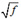, the p.u.l. resistance is growing also proportionally to. This qualitative result is practically very accurate, despite of the fact that the current density is not confined to the skin layer. This is also a kind of justification originally used for the skin depth term definition. The electric field cancellation in the conductor actually increases the current density in a thin layer close to the conductor surface (the charges are pushed to the surface and the total current does not change much, assuming that wave transfers the same power). That fact can be also used to explain the increase in the resistivity per unit length and decrease in the conductor interior inductance per unit length. With the current flowing in a thin layer, less magnetic field is located inside the conductor and thus less magnetic field energy is located inside the conductor (less internal inductance associated with that).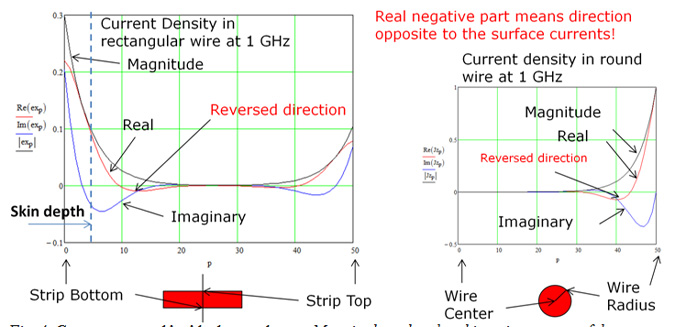Fig. 4. Current reversal inside the conductor. Magnitude and real and imaginary parts of the current density inside rectangular (numerical solution with reference conductor below the strip) and circular (exact analytical solution) conductors. The real part is the actual current.

If conductor surface is not flat and have small bumps (roughness), the absorption of the energy takes place at the conductor surface bumps (assuming that the bumps are much smaller than the wavelength of the propagative wave). The rough conductor surface will have simply more area to suck out more energy from the signal! As simple as that, but the quantification of this effect is relatively difficult and will be discussed later. The area increase on rough conductor can be substantial – two or more times for PCB and packaging conductors for instance. That means that the conductor losses may increase multiple times. We will discuss how it affects the signal propagation in details later. The effect can be called the skin effect on the rough surface. Though, all things considered, “suck-out effect” term would be actually more suitable instead of the “skin effect” in general J.

### Analysis of conductor effects on signal propagation

There are three major factors defining distribution of currents in the transmission line conductors and corresponding signal loss and dispersion. Those are conductor bulk resistivity, shape of the conductors and proximity of the conductors (strip and plane for instance). In addition surface roughness and dielectrics surrounding the conductors alter the current density in the interconnects that changes the p.u.l. impedance. The frequency-dependent conductor effects can be qualitatively separated into three frequency regions – low, medium and high [9,10]. This classification can be based on the state of current density distribution in conductors  or on the base of properties of the conductor impedance per unit length . Note that any classification is necessary only to understand the limitations of the models that include only some effects and do not take into account the others. Diagram in Fig. 5 provides qualitative illustration of the conductor effects that cause signal degradation (increase in attenuation or losses and phase distortion). The frequency axis on the diagram goes from top to bottom. The actual frequencies of the transitional states depends on the physical dimensions of the interconnects or scale and will be quantified later. The current is distributed uniformly in homogeneous trace and plane conductors from DC to some relatively low frequency (composite conductors have non-uniform current distribution even at DC). The corresponding impedance per unit length (p.u.l). has almost constant real part LDC and inductive part with almost constant inductance RDC that characterizes the uniform distribution of the electric field and current density inside both strips and planes. Note, that the conductive planes do not have shielding effect at these frequencies.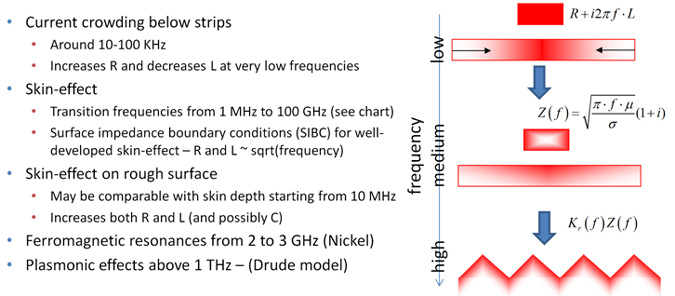Fig. 5. Current density changes with growth of frequency. At DC frequencies current is uniformly distributed over the strip and plane conductors. At relatively low frequency currents concentrates below the strip due to the proximity effect. As frequency increases or at the medium frequencies, current density becomes larger near surface of the conductors and at the strip edges. Finally, at high frequencies the skin effect is well-developed and roughness and dispersion plus edge effect may further contribute to the resistance growth and change the conductor interior p.u.l. inductance.

Current distribution in conductors starts changing at frequencies as low as 10 kHz for PCB case, where plane current concentrates below the strip to minimize the energy absorption by the plane (though these losses grow with the frequency). This first transition does not have much effect on the analysis of serial links and may be not so important to simulate – there are practically no signal harmonics below 100 kHz due to AC coupling capacitors used in the serial data links. At the medium frequency range, current density re-distribution inside the conductors takes place. Corresponding effects are not separable and usually called by different names like proximity or crowding effect, edge effect, transition to skin-effect. At medium frequencies the resistance p.u.l. increases and the internal conductor inductance p.u.l. decreases because of shrinking conductor area with the current and magnetic fields. Skin and edge effects become visible at higher frequencies and, finally, at high frequencies, most of the current flow in very thin surface layer and corresponding state can be characterized as the well-developed skin effect. Without the edges, dielectrics and proximity, the resistance at high frequencies grows proportionallyand conductor internal inductance decreases as 1/. Interaction of non-homogeneous dielectric dispersion and edge or proximity effects further accelerate the growth of resistance p.u.l. with frequency . This is valid even if very low loss or even ideal dielectrics are used. In addition, roughness causes a considerable increase the resistance p.u.l. at high frequencies [11-13].

The conductor shape and dielectric properties may have significant effect on the energy absorption in the conductors. Though, we can determine the medium or transition frequencies for different interconnect technologies that use strip or microstrip lines as illustrated in Fig. 6.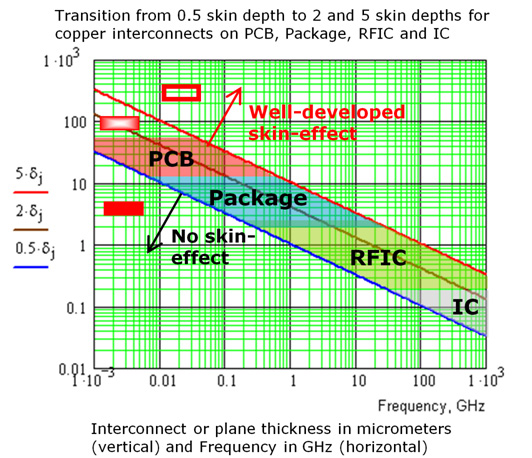Fig. 6. Transition frequencies for copper conductors. Strip or plane thickness in micrometers is the vertical axis and frequency in GHz is the horizontal axis. Strip thickness equal to 0.5 of skin depths and below (blue line) is considered as area with uniform current distribution (low frequencies). Strip thickness equal to 5 skin depths (red line) and higher is considered as area with well-developed skin-effect (high frequencies).

This can be done on the base of strip or plane thickness ratio to the skin depth at different frequencies. If conductor thickness is less or equal to 0.5δs (half skin-depth) the skin effect is practically not visible (uniform distribution of current density). We use it as the border of no skin effect area. For a particular trace thickness we can define the low frequency area – it is below the blue curve on the left graph in Fig. 6.  Frequency as high as 100 GHz can be considered low on the scale of the axis in Fig. 5 for interconnects with 0.1 um trace thickness or width. Simplified models without dispersion can be used at these frequencies for the analysis of integrated circuit interconnects. On the other end, the low frequency area falls into high kHz area for PCB interconnects. At frequencies when the strip thickness becomes equal to 2δs, the skin and edge effect become visible, but it is not well developed skin effect yet. According to rule of thumb in microwave engineering, the skin effect becomes well developed only if conductor thickness becomes equal to 0.5δs (five skin-depth rule). We will use it as the boundary for the well-developed skin-effect or high frequency area in Fig. 5. We can generalize this observation and define the transitional area or medium frequency range from 0.5δto 5δs. for different interconnect technologies as shown in Fig. 6. Strip or plane thicknesses on PCBs and in packages can range from 50 um to 1 um and corresponding transition frequencies may range from 1 MHz to above 10 GHz.

To illustrate the processes in and around the conductors, let’s use a symmetrical strip line with 7 mil wide 1.2 mil thick trace and two 1.2 mil thick planes at 8 mil distance above and below the strip (typical PCB trace). The strip and planes are made of copper. The strip is in low-loss homogeneous dielectric with dielectric constant 3.76 and loss tangent 0.006 at 1 GHz and simulated as the Wideband Debye or Djorjevic-Sarkar dielectric to account for the dielectric dispersion. The current density distributions in the strip and plane conductors at different frequencies without taking into account conductor surface roughness are computed with 3D full wave 3DTF solver in Simbeor THz software  and shown in Fig. 7-9. The pictures show peak of the current distribution as color map on logarithmic scale. 0 dB corresponds to the maximal current density value that increases with the frequency. The inserts on the right side of the pictures show peak current density distribution with arrows on linear scale. Skin depth values are shown on the pictures as sd and ratio of the conductor thickness to skin depth is also shown to feel the scale of the effect. Note, that the smallness of the skin layer at high frequencies makes it difficult even to visualize it. Triangulation is used in Fig. 7-9 and introduces some asymmetry on the color plots. Though, the arrow view on the inserts show that the current flow symmetrically on the top and bottom surfaces of the strip and in the maximal values of the current at the medium and high frequencies are observed in the corners (symmetry holds for the planes too).  Notice that the maximal value of the current density grows substantially with the frequency. At the same time, the total current in this numerical experiment was the same at all frequencies – approximately 0.01 Amperes (flows in the opposite direction in strip and on the planes).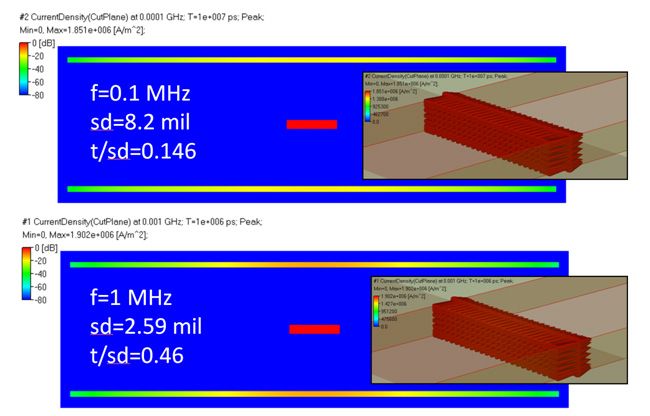Fig. 7. Current density distribution in strip and planes of symmetric strip line at low frequencies – current distribution is uniform on the strip and goes from almost uniform distribution in planes to the larger values below and above the strip.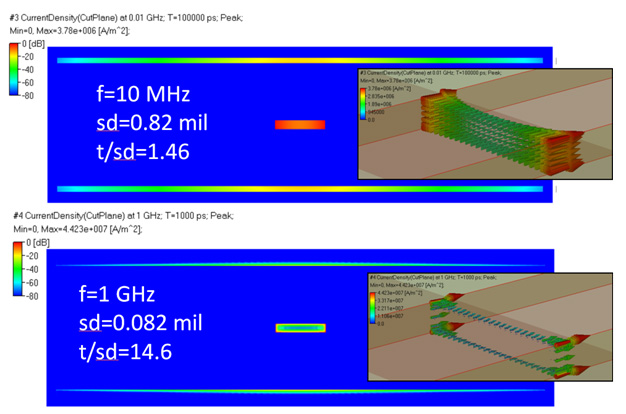Fig. 8. Current density distribution in strip and planes of symmetric strip line at the transition from low to high frequencies – current distribution first becomes non uniform on the strip (top picture) and finally flows in a thin layer on strip and plane surfaces.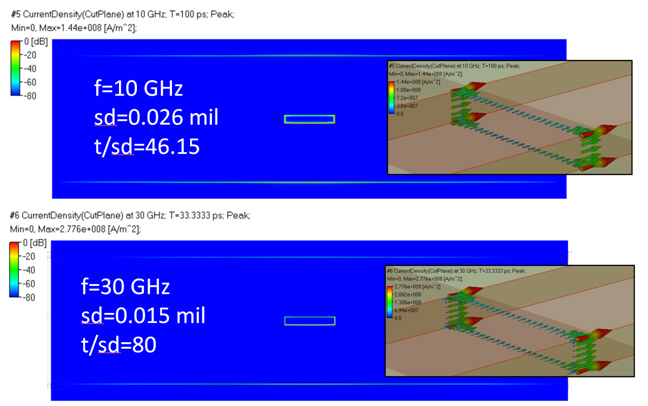Fig. 9. Current density distribution in strip and planes of symmetric strip line at high frequencies – current flows in extremely thin layer and higher current density is observed  in the conductor corners.

Power flow in the strip line cross section computed with Simbeor 3DTF solver at different frequencies is shown in Fig. 10. It basically illustrates the fact that the power is transferred in the dielectric between the strip and planes. The current crowding in planes and concentration at the edges changes the power flow distribution – more power flows closer to the strip (localization) and in the vicinity of the strip edges (the edge effect). At the same time, the power flow vectors inside the strip are pointed toward the strip interior as shown on the right inserts in Fig. 10. All vectors are normalized to the maximal power at a particular frequency and colored. The power flow associated with the conductor losses in strip line is very small comparing to the power flow through the cross-section at the frequencies up to 1 GHz. Thus, vectors lengths are not scaled on the pictures to see the power flow direction. The color of the vectors shows that the actual values are extremely small. At 30 GHz the power flow associated with the conductor losses becomes much larger – the vector lengths are scaled in this case. Notice that more power is absorbed on the strip edges – it corresponds to the larger values of the currents on the edges observed in Fig. 9.

See animation of the electromagnetic fields and instantaneous currents for this example in this YouTube video https://youtu.be/iys0de3Xq4E

Currents in microstrips are illustrated in Simberian app note #2015_01 and in this YouTube video https://youtu.be/epT8INlmCCg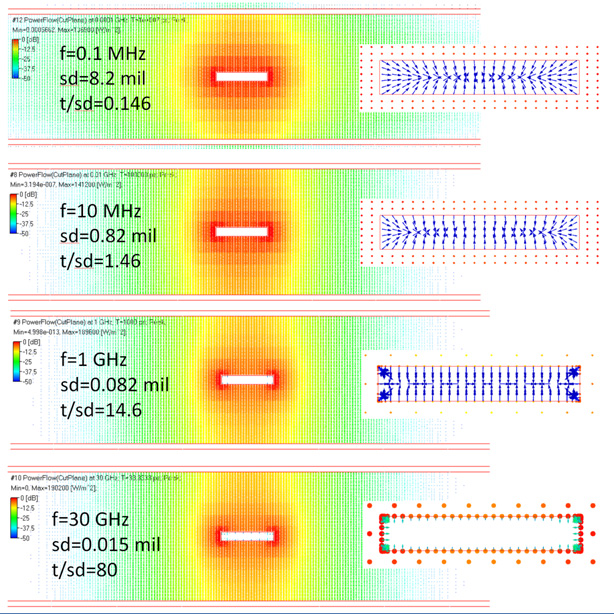Fig. 10. Power flow (peak value of Poynting vector) in strip line cross-section at different frequencies (logarithmic values ). The vectors are directed along the transmission line in area outside of the strip and plane conductors. Inside the conductor vectors are directed toward the conductor interior as shown for strip on the inserts (vectors are not scaled for 0.1, 10 MHz and 1 GHz for better visibility and scaled only for 30 GHz).

### Conductor surface roughness effect

Conductor surface roughness at high frequencies is another major contributor to the signal attenuation or degradation. Polishing of conductor and dielectric surfaces is not a possibility for the mass-production printed-circuit boards at this time. The roughness can increase the total interconnect loss as much as 50% and higher as shown in [11-13]. Actually, manufacturers of copper foils intentionally make surface rough to make it stick to dielectrics and harder to de-laminate. Technically, the roughness effect at PCB or packaging scale is simple increase of absorption by the non-flat conductor surface due to the increase of the conductor area exposed to the electromagnetic field. At lower frequencies, the roughness has effect only on the effective resistivity of the conductor (may increases it). But, as the frequency grows and the skin depth becomes comparable and then smaller than the bumps on the conductor surface and we observe the skin-effect on the non-flat conductor surface. Graph in Fig. 11 provides guidelines to determine at approximately what frequency the roughness has to be taken into account in the interconnect analysis. As soon as the roughness peak-to-valley root mean square (rms) value becomes comparable with the skin depth (0.5-1 of skin depth), it must be accounted for in the analysis. Some PCB manufacturing technologies have up to 10 um rms roughness (standard or reversed treated copper foil – STD or RTF) that starts degrading the signal at frequencies as low as 40 MHz. In contrast, modeling interconnects with rms roughness below 0.5 um (VLP, HVLP, ULP copper foil) may not require the roughness models at frequencies even below 5-10 GHz. The first conclusion from this observation is that making roughness bumps smaller reduces the loss associated with them.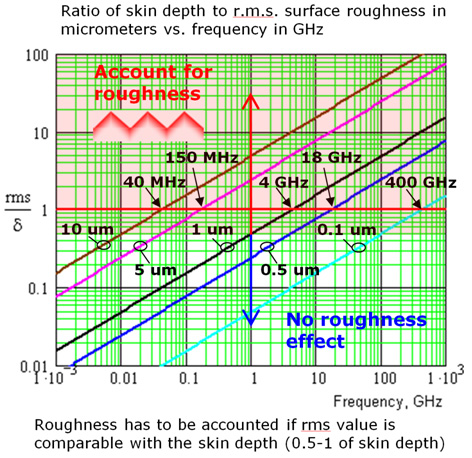Fig. 11. Ratio of rms surface roughness values to skin depth in copper: Brown line – 10 um, magenta – 5 um, black 1 um, blue 0.5 um, cyan – 0.1 um. Roughness must be taken into account in model if the ratio is about 0.5 or greater (shaded area).

Unfortunately, it is not so simple. Copper foil manufacturers try to make smaller bumps stickier and more adhesive to the dielectrics. Such surfaces have a lot of structure (see example in Fig. 12) that increases the absorption area (technically it is effective absorption length for the quasi-TEM waves, with the electric field in conductor oriented along the wave propagation) and may substantially increase the conductor absorption and corresponding losses at high frequencies.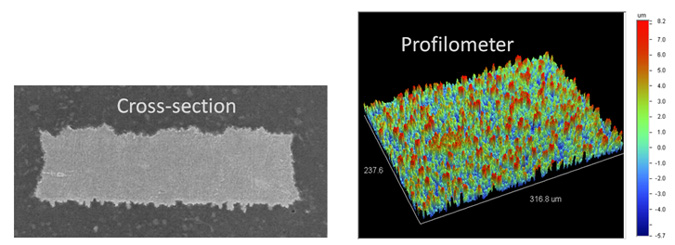Fig. 12 Examples of rough conductor surfaces from micro-photograph of a strip line cross-section and measured with profilometer .

The complexity of the rough conductor surface as illustrated in Fig. 12 is what makes the roughness modeling particularly difficult. Measurements with profilometer do not provide all the details of the surface, visible on the micro-photographs and use of such measurements for the model construction is very limited. In general, it is difficult to quantify the roughness effect using just the measurement of physical structure of the surface [12,13,15].

The conductor roughness modeling is the area of ongoing research – see overview in . Direct electromagnetic analysis of such surfaces is simply out of question – even the structure of the surface is unknown to start with. It would be totally non-practical approach even if we properly “scan” the surface geometry. We will discuss just two approaches suitable for practical purpose.

The first approach is to model roughness with an “effective” material layer. The mixture of conductor and dielectric on the conductor surface can be modelled as a layer of material with some “effective” properties. In general, such layer can be either conductive or dielectric or mixture of both. The “effective” roughness dielectric approach was introduced in  and illustrated in Fig. 13.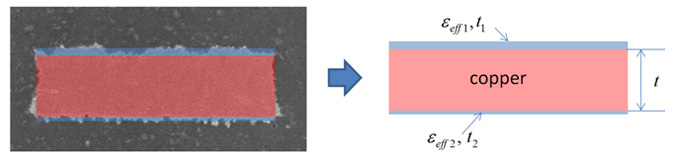Fig. 13 Effective roughness dielectric approach for conductor surface roughness modelling. Thin layers with mixture of conductor and dielectric on the conductor surface are turned into thin layers of dielectric with effective permittivity in the model.

There is less uncertainty in the conductor to dielectric boundary definition in this approach – the boundary is replaced with a transitional layer. On the other hand, the model has too many parameters. For instance, type of dielectric model for a particular type of surface roughness and parameters of such model are not readily available in copper foil spreadsheets.

The second approach is to use roughness correction coefficients in transmission line or electromagnetic models. This approach is based on the estimation of increase in attenuation with the frequency due to conductor surface non-flatness with a formula with one or more parameters in addition to frequency. Probably, the first and the simplest .roughness correction coefficient (RCC) is so called Hammerstad-Jensen model . It was derived for conductor surface with 60-degree triangular bumps and extensively used in microwave applications to evaluate the increase in attenuation of strip and microstrip lines. The main disadvantage of the model is that the maximal value of the expected increase in attenuation was two. That is not a problem for the microwave applications, where the skin depth is usually much larger than the surface roughness bumps (they use manufacturing technology with almost no roughness). This is not the case for the PCB and packaging applications, where surface bumps may be comparable and often larger than the skin depth. To overcome the limitation of the factor of two, Modified Hammerstad RCC (MHRCC) was recently introduced in [12,13] and is illustrated in Fig. 14. The roughness correction coefficient Ksr has two parameters: Δ or Surface Roughness (SR) parameter is approximately root mean square peak-to-valley measurement of roughness bumps, and RF is roughness factor that defines the maximal expected growth of losses due to roughness (increase of the surface area or effective absorption area or length on the rough surface). Ksr is unit at lower frequency (no increase in attenuation), and grows with the frequency as illustrated on the graph in Fig. 14 for Δ = 1 micron. RF=2 corresponds to the original Hammerstad-Jensen model with 60 degree triangular bumps (red line) and predicts increase in the attenuation by factor of 2.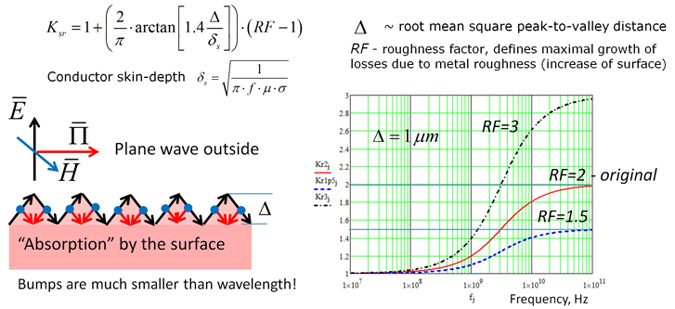Fig. 14 Modified Hammerstad roughness correction coefficient. The formula describes increase in attenuation of plane wave propagating along the conductor surface with triangular bumps. Power flow vector of the plane wave is directed along the surface, but the power flows into the conductor on the bumps (red arrows).

Surfaces with larger values of SR increase the attenuation at lower frequencies. Though, such surfaces may have larger values of RF and predict more losses at higher frequencies. Conductors with low profile roughness in PCB applications may increase the attenuation by more than 2 times (RF>2). Fig. 14 also illustrates the power absorption process that explains the physics of the model.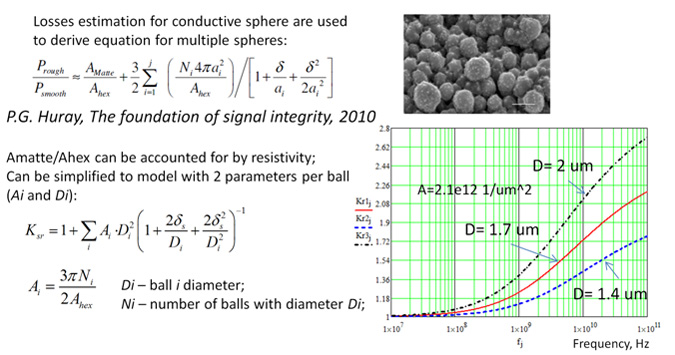Fig. 15 Huray snowball roughness correction coefficient. The original power loss estimation formula (top formula) and simplified model with 2 parameters per ball (Ksr, bottom formula).

To estimate losses on the rough conductor surface, Paul Huray  solved problem of plane wave diffraction on a conductive sphere and use it to evaluate the power loss for multiple spheres as illustrated in Fig. 15. The reasoning for such approach was the observation that some conductor treatment processes produce surfaces that look like a bunch of snow-balls such as shown on the micro-photograph in Fig. 15. The model is not a solution for a bunch of connected spheres, but can be used as the first approximation. The original power loss formula can be turned into the RCC with two parameters per one ball as shown in Fig. 15. As with the MHRCC, the Huray’s Snowball RCC (HSRCC) has values close to unit at lower frequencies and grow with the frequency as illustrated by graph in Fig. 15. Balls with larger diameter produce larger attenuation increase at high frequencies.

Both MHRCC and HSRCC models have “physical” explanation or background, but it is usually either difficult or simply not possibly to link the geometry of the conductor surface to the model parameters. The geometry of the conductor surface is usually not known to start with. Though, both MHRCC and HSRCC can be simply treated as parametric models and parameters just fitted to match measured insertion loss or attenuation [19,20]. The reasoning here is the same as with the causal models of dielectrics such as Debye model – as engineers, we do not care what particular atoms or molecules produced  the relaxation for a particular Debye term. We can just fit it to measurements and start using.

Considering the application of the roughness correction coefficients to the analysis of interconnects, there are three options here as illustrated in Fig. 16.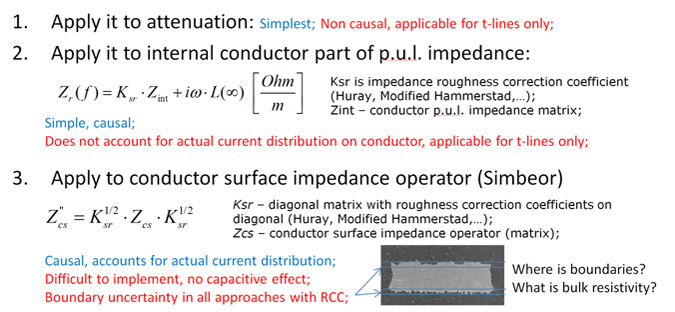Fig. 16. Use of roughness correction coefficients in the analysis of roughness effect on signal propagation

All roughness correction coefficients (Huray’s snowball, Modified Hammerstad, hemispherical model, Sandstroem’s model, stochastic models, - see overview in ) were originally used simply as the additional factor for transmission line attenuation, to evaluate the losses increase (item 1 in Fig. 16). This is simplest approach, but in the context of interconnect analysis can be used only for crude evaluation of the attenuation increase in the channel. If just attenuation is increased in a t-line model, such model is not causal. With a static or quasi-static field solver used for the analysis of t-line cross-section, RCC can be used to correct the internal impedance part of the total p.u.l. impedance of the transmission line as shown at item 2 in Fig. 16. This approach is causal because of it modifies both real and imaginary parts of the conductor impedance. However, correction of p.u.l. impedance does not accounts for the actual distribution of the current in the conductors. As we see from Fig. 8 and 9, currents on the conductor edges can be much larger. Also, currents on top and bottom side of a microstrip line can substantially different. Current distribution is also different for different t-line modes. All that leads to differences in the observed attenuation growth with the frequency. To account actual current distribution on a conductor, the roughness correction coefficients has to be applied in the numerical electromagnetic model locally at the boundaries between the conductor and dielectric as illustrated at item 3 in Fig. 16. For instance, if interior of a conductive material is described with the impedance matrix or impedance operator, it can be simply adjusted by multiplying it by two matrices with square root of the RCC on the diagonals as suggested in [12,13]. Technically, such transformation corresponds to connection of ideal transformers with the transformation coefficients equal to square roots of RCC on the boundary between Trefftz’s finite elements inside and outside the conductor. This approach can be easily implemented in approaches that use the immittance or scattering parameters to solve electromagnetic problems . The approach is causal and accounts for the actual current distribution, but it has the uncertainty of the conductor to dielectric boundary definition. There must be an procedure to define the boundary as proposed in . The boundary positioning affects the “effective” conductor resistivity and also capacitance and thus the characteristic impedance. All those effects are tightly related in context of the interconnect models, where we need to have analysis to measurement correlation starting from 1 MHz up to 100 GHz. It may be not so important for the analysis of the narrow-band microwave circuits with polished conductors.

The simplest way to demonstrate how roughness affects the signal propagation is to use a semi-analytical waveguide model, such as parallel-plate waveguide as shown in Fig. 17. It has two conductive plates and Perfect Magnetic Conductor (PMC) boundary conditions on the sides. The dominant wave of such waveguide is pure TEM wave, and in case of the lossy conductor it is quasi-TEM wave due to longitudinal electric field on the lossy conductor surface (similar to PCB interconnects).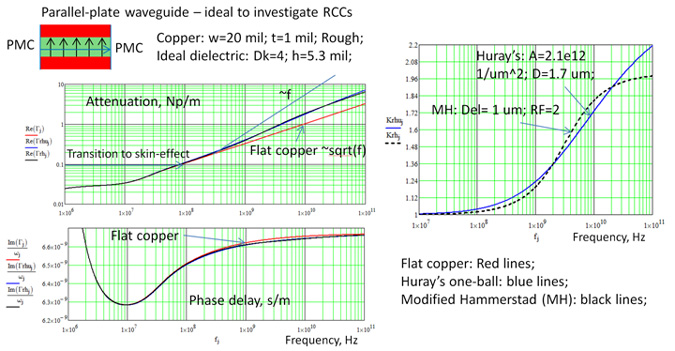Fig. 17. Roughness effect on attenuation (top left plot) and phase delay (bottom left plot) in parallel plate waveguide. Red line – no roughness, black and blue lines – conductors with surface impedance adjusted with HSRCC and MHRCC defined on the right.

With flat copper conductors or without roughness, the attenuation in such waveguide grows proportionally to square root of frequency at the well-developed skin effect bandwidth, as can be predicted by the conductor interior model shown in Fig. 3. The attenuation and phase delay per meter vs. frequency are shown in Fig. 17 by red lines on the left plots. The current distribution in the parallel-plate waveguide is uniform – no edge or proximity effects. To investigate how conductor surface roughness can change the attenuation and phase delay, two roughness correction coefficients are used – HSRCC and MHRCC with the parameters and frequency dependency shown on the right plot of Fig. 17. Both model produce similar very close increase in the attenuation as shown in Fig. 17. We can observe that the attenuation with rough conductors grows between the square root (flat conductor) and the linear frequency dependency (typical for dielectrics). Though, there is not much effect on the phase delay. This is because the conductor internal inductance adjustment is small comparing to the total p.u.l. inductance. Scott McMorrow suggested and demonstrated how to use this fact to separate the conductor roughness and dielectric losses .

Finally, let’s compare the effective roughness dielectric (ERD) model with the model with roughness correction coefficients. We use the same strip line that was investigated in Fig. 7-9 with 7 mil wide 1.2 mil thick trace and two 1.2 mil thick planes at 8 mil distance above and below the strip. The strip and planes are made of copper. The strip is in low-loss homogeneous dielectric with dielectric constant 3.76 and loss tangent 0.006 at 1 GHz and simulated as the Wideband Debye or Djorjevic-Sarkar. Parameters of the ERD layers are shown in Fig. 18 and were identified and validated with the measurements for standard or STD copper in . Wideband Debye model is used for the ERD models with dielectric constant (DK) and loss tangent (LT) parameters shown in Fig. 18 applied to 1 GHz.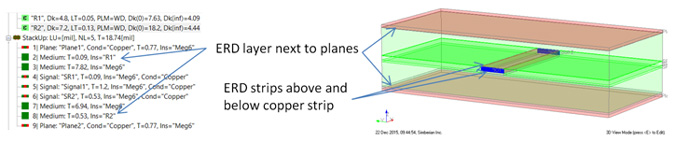Fig. 18. Effective roughness dielectric use for the strip line analysis in Simbeor THz software. Bottom of the top plane and top of the strip are ”oxide” sides modeled with T1=2.27 um, DK=4.8 and LT=0.05. Top of the bottom plane and bottom of the strip surface are “foil” sides modeled with T2=13.5 um, DK=7.2 and LT=0.13.

Model with the roughness correction coefficient have the cross-section of the original strip line. MHRCC is used with SR=0.25 um and RF=3 for “oxide” and SR=0.5 um and RF=6 for the “foil” sides of strip and planes.

Transmission line attenuation and characteristic impedance computed without roughness and with two roughness models with Simbeor 3DTF solver are shown in Fig. 19. The modal strip line parameters are used to compute insertion loss and phase delay in 15.41 inch segment of strip line plotted in Fig. 20. Both models with roughness predict substantial increase in the attenuation or insertion loss as expected. Though, the model with RCC predicts slight increase in the characteristic impedance due to increase of the conductor internal inductance on the rough surface. ERD predicts decrease in the characteristic impedance due to higher dielectric constant of the ERD layers and additional capacitance associated with that. The capacitive effect of the roughness for STD copper was previously noticed in [12,13] and was attributed to sharp peaks on the surface of the copper foil as was previously suggested in . The increase in the capacitance may be achieved with the transitional layer with larger dielectric constant as in ERD model (different reason of the increase). If the effect is real and actual board shows smaller impedance, the boundaries of the conductors in the models with the roughness correction coefficients may be also adjusted to account for the effect (stackup adjustment). There are no esteblished methods for such adjustments.

Increase both in the internal conductor inductance predicted by MHRCC and in p.u.l. capacitance predicted by ERD model cause the increase in the phase delay as shown on the right plot in Fig. 20. Again, ERD predicts larger increase due to larger adjustment of the capacitance that can be accounted for in the MHRCC model with the cross-section geometry correction. Electromagnetic fields, currents and power flow were also computed and animated for better understanding of the roughness models – see YouTube videos https://youtu.be/mx3_H0olIf8 (ERD) and https://youtu.be/PsM2Wu0pkRo (MHRCC).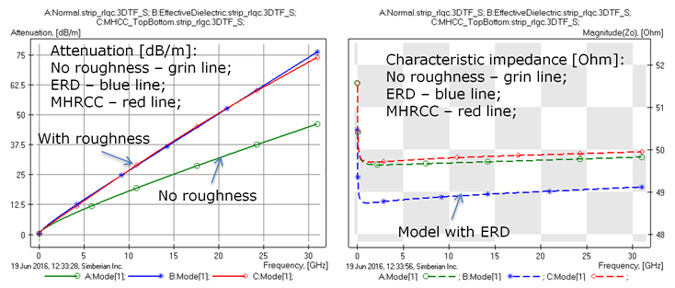Fig. 19. Strip line attenuation (left plot) and characteristic impedance (right plot) computed without roughness (grin lines) with ERD model (blue lines) and with MHRCC model (red lines).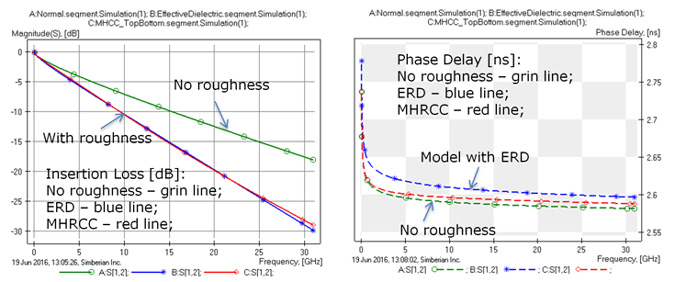Fig. 20. Insertion loss (left plot) and phase delay (right plot) in 15.41 in strip line segment computed without roughness (grin lines) with ERD model (blue lines) and with MHRCC model (red lines).

### Ferromagnetic conductors

All materials in general and conductors in particular have some magnetic properties or non-unit magnetic permeability. All conductors exhibit some amount of magnetic polarization when external magnetic field is applied. Though, only ferromagnetic metals have permeability that may be substantially larger than unit and have to be accounted in the electromagnetic analysis. Cobalt, iron and nickel are examples of ferromagnetic conductors. Only nickel is often used in manufacturing of PCB and packaging interconnects in surface coating techniques such as ENIG and ENEPIG. As was demonstrated in , ferromagnetic properties of nickel can cause substantial signal degradation if interconnects are finished with the ENIG process. This is due to larger bulk resistivity of nickel and non-unit permeability. As we can see from the skin depth formula shown in Fig. 3, both can reduce the skin depth and, thus, increase the p.u.l. resistance of the conductor. This is in addition to increase in the internal conductor p.u.l. inductance. In addition to the higher resistivity and permeability, nickel layer may exhibit resonance at the relatively low frequencies between 2 and 3 GHz. Landau-Lifshits or Lorentz models can be effectively used to simulate the resonance as illustrated in Fig. 21. The plot on the right shows real and imaginary parts of the permeability with the parameters identified for ENIG process in [Scott and I]. As shown in Fig. 21, the resonance can be observed in the measurements as increase of insertion losses and dispersion of group delay around 2.7 GHz. All that can substantially degrade the signal with frequency harmonics at those freuqencies.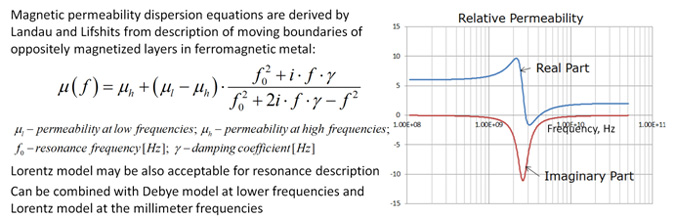Fig. 21. Modeling ferromagnetic properties of nickel with Landau-Lifshits model.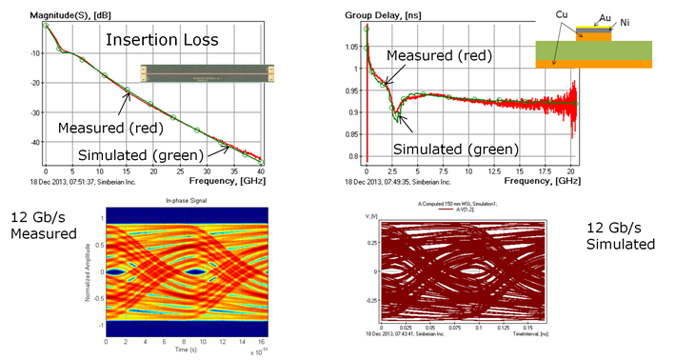Fig. 21. Effect of nickel plating on packaging interconnect – comparison of measured and simulated insertion loss (top left), group delay (top right) and eye diagrams (measured – bottom left, simulated – bottom right).

### Beyond the skin-effect

Theory of the conductor bulk conductivity or resistivity was first developed by Paul Drude . Drude used classic theory of electrons in a “pinball” machine and derived Ohm’s low in the form shown in Fig. 1 and Fig. 22. The theory shows that the reaction of the electrons in conductors has very small delay when the external electric field is applied. The delay is insignificant up to THz frequency range. Constant bulk resistivity or conductivity corresponds to the delay-less conductor model that we usually use to simulate the conductors.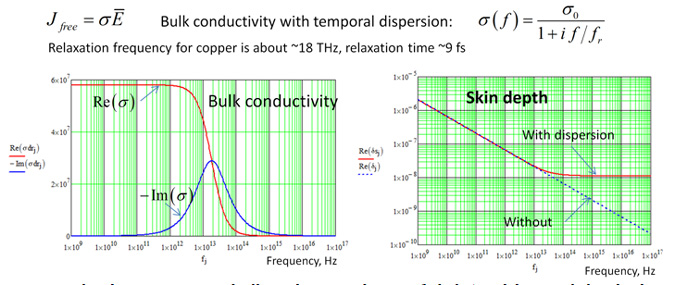Fig. 22. Real and imaginary part of sulk conductivity of copper (left plot) and the actual skin depth in copper predicted by dispersive model (red line) and by the constant conductivity model (blue dashed line).

However, as frequency grows the delay has to be taken into account. The temporal relaxation processes in conductors are non-resonant and with high accuracy can be described by the exponential relaxation or by Drude model as illustrated in frequency domain in Fig. 22. The model is very similar to Debye model for dielectric polarization, because of both models describe exponential relaxation responses of the conductor and dielectric materials. σ0 in formula shown in Fig. 22 is the bulk conductivity of annealed copper 5.8*10^7 S/m. Drude theory was later corrected and extended by Hendrik Antoon Lorentz (Dutch) and Arnold Sommerfeld. The classic model was replaced by theory that described conductor behavior as electron gas similar to gas of molecules or to plasma. Electrons in metals behave as plasma and can form electron density waves or plasmons. Though, the electron density waves can be accurately described with the original relaxation Drude model for the conductivity. More often the equivalent model with the complex conductor permittivity is used to explain the plasmonic effects. One of the interesting consequences of the electron plasma relaxation model is the saturation or the end of the skin effect as illustrated on the right plot in Fig. 22 for copper. Metals became practically transparent to electromagnetic fields above the plasma frequency. Starting from some frequency in the infrared frequency range, the skin depth does not decrease with the frequency as predicted by the model with the constant conductivity or without the dispersion. It means that interconnects at nanometer scale will never have skin effect at all frequencies – the skin depth will be always larger than the cross-section, even at the optical frequencies! Plasmonic behavior of conductors at high frequencies can be used to create new types of interconnect structures such as surface plasmon-polaritron waveduides that require just one wire to transmit the energy. This is a viable alternative to optical interconnects based on dielectric materials that does not scale down well due to some fundamental limitations such as bending losses and the diffraction limit. Though, this is usable only on IC scale and well beyond of the PCB and packaging interconnects subject.

### Conclusion

The paper provides broad overview of the conductor effects on the signal propagation in PCB and packaging interconnects. After reading this paper you should be able to understand what is going on in the conductors from DC to daylight frequencies. From the macroscopic electromagnetics point of view, the conductors are very simple materials – described by the Ohm’s law with constant bulk conductivity up to THz frequency range. This is the model with just 1 parameter – conductivity or resistivity that can be easily measured at DC and used up to THz frequency range! Though, the scale of the skin depth changes and complexity of the rough surfaces may complicate the picture and make the problem difficult for the numerical analysis. Conductor roughness models with effective roughness dielectric or with the local adjustment of the conductor surface impedance with the roughness correction coefficients are not available in most of the EDA tools. Simplified one-parameter roughness models or use of static field solvers may be too approximate and substantially increase risks at the data rates above 10 Gbps. The conductor models must be validated with the measurements in the frequency range of the signal spectrum.

Finally, if we want to move toward 100 Gbps, here is the top three requirements for the interconnect analysis software:

1. Extraction of p.u.l. and modal transmission line parameters with quasi-static field solver for strip lines or with  3D full-wave analysis for microstrip lines;
2. Have conductor interior models valid and causal at least over 5-6 frequency decades in general to account for the current crowding, proximity and edge effects;
3. Simulate conductor surface roughness with at least two-parameter models such as modified Hammerstad or Huray’s snowball model;

### References

1.      Y. Shlepnev, Sink or swim at 28 Gbps, The PCB Design Magazine, October 2014, p. 12-23 - the original version is also available here.

2.      C.T.A. Johnk, Engineering electromagnetic – Fields and waves, J. Wiley & Sons, 1975.

3.      C.A. Balanis, Advanced engineering electromagnetics, J. Wiley & Sons,  2012.

4.      I. S Rez, Y.M. Poplavko, Dielectrics (in Russian), Radio i Sviaz’, 1989.

5.      Y. Shlepnev, Decompositional Electromagnetic Analysis of Digital Interconnects, IEEE International Symposium on Electromagnetic Compatibility (EMC2013), Denver, CO, 2013, p. 563-568,

7.      C.R. Paul, Analysis of multiconductor transmission lines, J. Wiley & Sons, 1994.

8.      F. Olyslager, Electromagnetic waveguides and transmission lines, Oxford, 1999.

9.      A. R. Djorjevic, T. K. Sarkar, Closed-form formulas for frequency-dependent resistance and inductance per unit length of microstrip and strip transmission lines, IEEE Trans. Microwave Theory Tech, vol. 42, N 2, 1994, p. 241-248.

10.  J. Rautio, An Investigation of Microstrip Conductor Loss, IEEE MTT Magazine, December 2000, pp. 60 – 67.

11.  A. Deutsch, C Surovic, R. Krabbenhoft, G. V. Kopcsay, B. Chamberlin, Losses caused by roughness of metallization in printed-circuit boards, - in Proc. of 14th Topical Meeting on Electrical Performance of Electronic Packaging, 2005, p. 39-42.

12.  Y. Shlepnev, C. Nwachukwu, Roughness characterization for interconnect analysis. - Proc. of the 2011 IEEE International Symposium on Electromagnetic Compatibility, Long Beach, CA, USA, August, 2011, p. 518-523.

14.  Simbeor THz – Electromagnetic Signal Integrity Software, Simberian Inc., www.simberian.com.

15.  A.V. Rakov, S. De, M.Y. Koledintseva, S. Hinaga, J.L. Drewniak, R.J. Stanley, Quantification of conductor surface roughness profiles in printed circuit boards, IEEE Trans. on EMC, v. 57, N2, 2015, p. 264-273.

16.  M.Y. Koledintseva, A. Ramzadze, A. Gafarov, S. De, S. Hinaga, J.L. Drewniak, PCB conductor surface roughness as a layer with effective material parameters. – in Proc. IEEE Symp. Electromagn. Compat., Pittsburg, PA, USA, 2012, p. 138-142.

17.  E.O.  Hammerstad,  Ø.  Jensen,  “Accurate  Models  for  Microstrip  Computer  Aided  Design”, IEEE MTT-S Int. Microwave Symp. Dig., p. 407-409, May 1980.

18.  P. G. Huray, The foundations of signal integrity, IEEE and J. Wiley & Sons, 2010.

19.  Y. Shlepnev, Broadband material model identification with GMS-parameters, in Proc. of 2015 IEEE 24st Conference on Electrical Performance of Electronic Packaging and Systems, October 25-28, 2015, San Jose, CA.

21.  Y.O. Shlepnev, "Trefftz finite elements for electromagnetics", - IEEE Trans. Microwave Theory Tech., vol. MTT-50, pp. 1328-1339, May, 2002.

23.  A. Albina at al., Impact of the surface roughness on the electrical capacitance, Microelectron. J. 37 (2006) 752-758.

24.  Y. Shlepnev, S. McMorrow, Nickel characterization for interconnect analysis. - Proc. of the 2011 IEEE International Symposium on Electromagnetic Compatibility, Long Beach, CA, USA, August, 2011, p. 524-529.

### Appendix 1: Maxwell’s Equations

Macroscopic form of Maxwell’s equations without material or constitutive equations in SI units: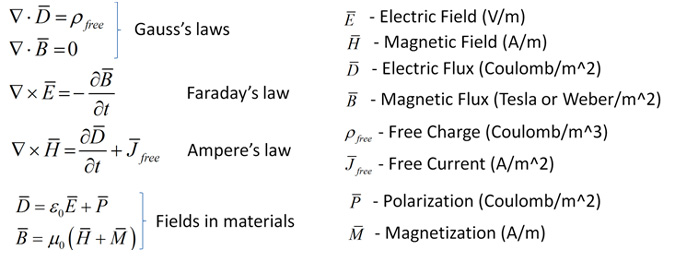### Appendix 2: Definitions

Dielectrics are the broad expanse of nonmetals considered from the standpoint of their interactions with electric, magnetic or electromagnetic fields. - A. R. von Hippel, “Dielectric materials and applications”.

Conductors are materials that allow the flow of electrical current.

Conductors are also elements of interconnects made of conductive materials.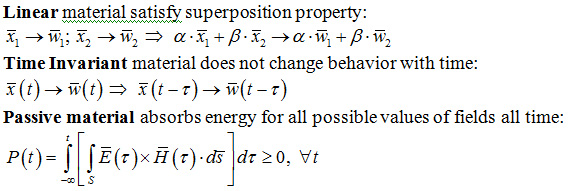Material is homogeneous if properties do not change through some area/volume.

Material is isotropic if properties do not change with direction.

Material is anisotropic if properties change with direction.

Temporal dispersion is momentary delay or lag in properties of a material usually observed as frequency dependency of the material properties.

p.u.l. – per unit length parameters of a transmission line.

RCC – roughness correction coefficient.

HSRCC – Huray’s snowball roughness correction coefficient.

MHRCC – modified Hammerstad roughness correction coefficient.

ERD – effective roughness dielectric.